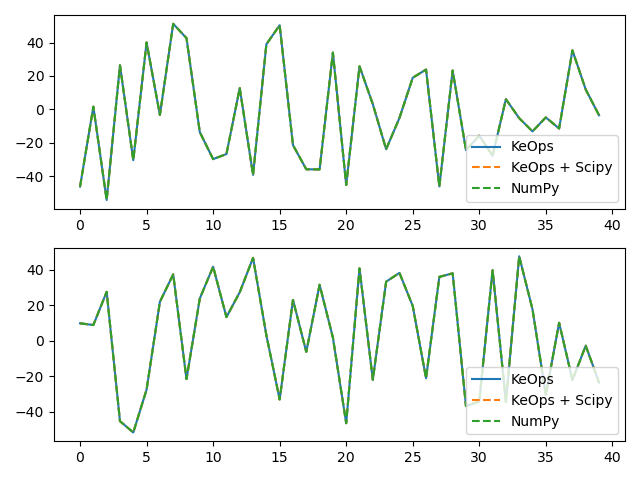# KernelSolve reduction (with LazyTensors)¶

Let’s see how to solve discrete deconvolution problems using the conjugate gradient solver provided by the pykeops.numpy.LazyTensor.solve() method of KeOps pykeops.numpy.LazyTensor.

## Setup¶

Standard imports:

import time

import matplotlib.pyplot as plt
import numpy as np

from pykeops.numpy import Vi, Vj, Pm
from pykeops.numpy.utils import IsGpuAvailable


Define our dataset:

N = 5000 if IsGpuAvailable() else 500  # Number of points
D = 2  # Dimension of the ambient space
Dv = 2  # Dimension of the vectors (= number of linear problems to solve)
sigma = .1  # Radius of our RBF kernel

x = np.random.rand(N, D)
b = np.random.rand(N, Dv)
g = np.array([.5 / sigma ** 2])  # Parameter of the Gaussian RBF kernel

alpha = 0.01


Apply our solver on arbitrary point clouds:

print("Solving a Gaussian linear system, with {} points in dimension {}.".format(N, D))
start = time.time()
Kxx = (-Pm(g) * Vi(x).sqdist(Vj(x))).exp()
c = Kxx.solve(Vi(b), alpha=alpha)
end = time.time()
print('Timing (KeOps implementation):', round(end - start, 5), 's')


Out:

Solving a Gaussian linear system, with 5000 points in dimension 2.
Timing (KeOps implementation): 4.10121 s


Note

The pykeops.numpy.LazyTensor.solve() method uses a conjugate gradient solver and assumes that Kxx defines a symmetric, positive and definite linear reduction with respect to the alias "b" specified trough the third argument.

Apply our solver on arbitrary point clouds:

Compare with a straightforward Numpy implementation:

start = time.time()
K_xx = alpha * np.eye(N) + np.exp(- g * np.sum((x[:, None, :] - x[None, :, :]) ** 2, axis=2))
c_np = np.linalg.solve(K_xx, b)
end = time.time()
print('Timing (Numpy implementation):', round(end - start, 5), 's')
print("Relative error = ", np.linalg.norm(c - c_np) / np.linalg.norm(c_np))

# Plot the results next to each other:
for i in range(Dv):
plt.subplot(Dv, 1, i + 1)
plt.plot(c[:40, i], '-', label='KeOps')
plt.plot(c_np[:40, i], '--', label='NumPy')
plt.legend(loc='lower right')
plt.tight_layout();
plt.show()Out:

Timing (Numpy implementation): 1.38177 s
Relative error =  4.6076718316301283e-07
/home/bcharlier/keops/pykeops/examples/numpy/plot_test_invkernel_numpy_helper.py:81: UserWarning: Matplotlib is currently using agg, which is a non-GUI backend, so cannot show the figure.
plt.show()


Total running time of the script: ( 0 minutes 16.544 seconds)

Gallery generated by Sphinx-Gallery